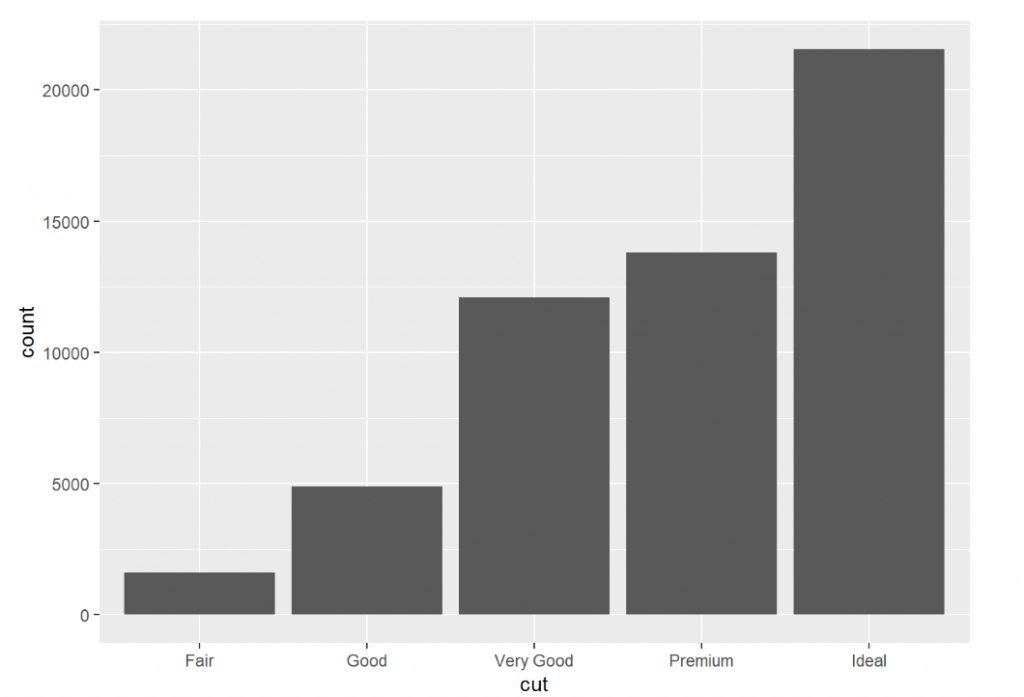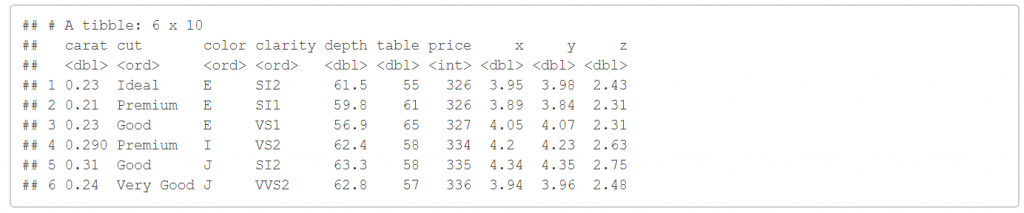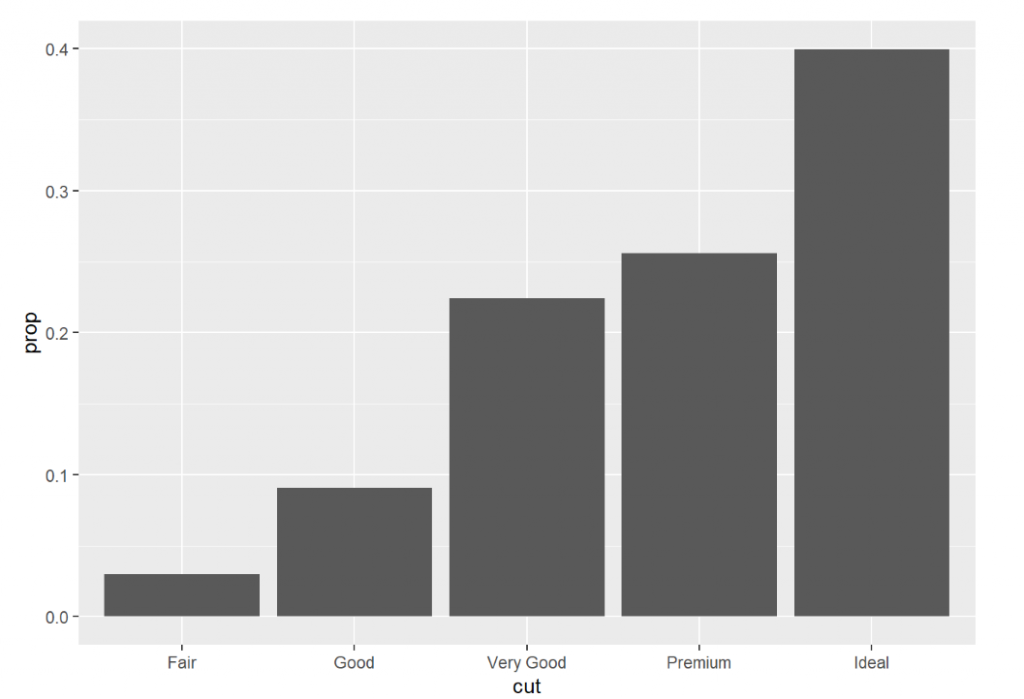#0

## [R語言]資料分析讀書心得04---geom and stat

Computed variables:

1.count:number of points in bin

2.prop:groupwise proportion

``````library(tidyverse)
``````

ggplot(data=diamonds)+
geom_bar(mapping=aes(x=cut,y=color))

Run `rlang::last_error()` to see where the error occurred.

``````ggplot(data=diamonds)+
geom_bar(mapping=aes(x=cut))
````````````head(diamonds)
````````````ggplot(data=diamonds)+
geom_bar(mapping=aes(x=cut,y=..prop..,group=1))
``````statgeom 的關係其實相當複雜，不過這篇文章的介紹主要是希望大家可以稍微了解圖形的畫法，今天的介紹到這，謝謝大家。